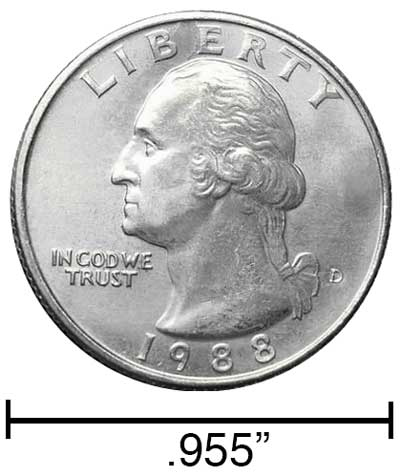# Kilometers to Inches Conversion

Enter the length in kilometers below to get the value converted to inches.

Results in Inches:1 km = 39,370.07874 in
1 km ≈ 39,370 5/64"

## How to Convert Kilometers to InchesTo convert a kilometer measurement to an inch measurement, multiply the length by the conversion ratio.

Since one kilometer is equal to 39,370.07874 inches, you can use this simple formula to convert:

inches = kilometers × 39,370.07874

The length in inches is equal to the kilometers multiplied by 39,370.07874.

For example, here's how to convert 5 kilometers to inches using the formula above.
5 km = (5 × 39,370.07874) = 196,850.393701"

Our inch fraction calculator can add kilometers and inches together, and it also automatically converts the results to US customary, imperial, and SI metric values.

## Kilometers

One kilometer is equal to 1,000 meters, which are defined as the distance light travels in a vacuum in a 1/299,792,458 second time interval.

The kilometer, or kilometre, is a multiple of the meter, which is the SI base unit for length. In the metric system, "kilo" is the prefix for 103. Kilometers can be abbreviated as km; for example, 1 kilometer can be written as 1 km.

## Inches

An inch is a unit of linear length measure equal to 1/12 of a foot or 1/36 of a yard. Because the international yard is legally defined to be equal to exactly 0.9144 meters, one inch is equal to 2.54 centimeters.

The inch is a US customary and imperial unit of length. Inches can be abbreviated as in; for example, 1 inch can be written as 1 in.

Inches can also be denoted using the symbol, otherwise known as a double-prime. Often a double-quote (") is used instead of a double-prime for convenience. A double-prime is commonly used to express 1 in as 1″.The standard ruler has 12", and is a common measuring tool for measuring inches. They are also often measured using tape measures, which commonly come in lengths from 6' - 35'. Other types of measuring devices include scales, calipers, measuring wheels, micrometers, yardsticks, and even lasers.

We recommend using a ruler or tape measure for measuring length, which can be found at a local retailer or home center. Rulers are available in imperial, metric, or combination with both values, so make sure you get the correct type for your needs.

Need a ruler? Try our free downloadable and printable rulers, which include both imperial and metric measurements.

## Kilometer to Inch Conversion Table

Kilometer measurements converted to inches
Kilometers Inches
0.0001 km 3.937"
0.0002 km 7.874"
0.0003 km 11.81"
0.0004 km 15.75"
0.0005 km 19.69"
0.0006 km 23.62"
0.0007 km 27.56"
0.0008 km 31.5"
0.0009 km 35.43"
0.001 km 39.37"
0.002 km 78.74"
0.003 km 118.11"
0.004 km 157.48"
0.005 km 196.85"
0.006 km 236.22"
0.007 km 275.59"
0.008 km 314.96"
0.009 km 354.33"
0.01 km 393.7"
0.02 km 787.4"
0.03 km 1,181"
0.04 km 1,575"
0.05 km 1,969"
0.06 km 2,362"
0.07 km 2,756"
0.08 km 3,150"
0.09 km 3,543"
0.1 km 3,937"
0.2 km 7,874"
0.3 km 11,811"
0.4 km 15,748"
0.5 km 19,685"
0.6 km 23,622"
0.7 km 27,559"
0.8 km 31,496"
0.9 km 35,433"
1 km 39,370"

## References

1. Ambler Thompson and Barry N. Taylor, Guide for the Use of the International System of Units (SI), National Institute of Standards and Technology, https://physics.nist.gov/cuu/pdf/sp811.pdf
2. National Institute of Standards and Technology, Checking the Net Contents of Packaged Goods, Handbook 133 - 2019 Edition, https://nvlpubs.nist.gov/nistpubs/hb/2019/NIST.HB.133-2019.pdf